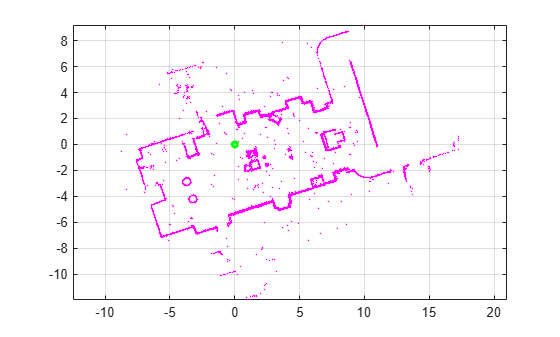# findPose

Find absolute pose of 2-D lidar scan in the map

Since R2022b

## Syntax

``absPose = findPose(scanMapObj,scan)``
``absPose = findPose(scanMapObj,scan,positionEstimate)``
``[absPose,scanID,score] = findPose(___)``
``[___] = findPose(___,Name=Value)``

## Description

example

````absPose = findPose(scanMapObj,scan)` finds the absolute pose for a scan in the map matching the input scan.```
````absPose = findPose(scanMapObj,scan,positionEstimate)` specifies a position estimate for the input scan with respect to the map. This reduces the computation time of the function.```
````[absPose,scanID,score] = findPose(___)` returns the scan ID of the matching scan and the corresponding correlation score, using any combination of input arguments from previous syntaxes.```
````[___] = findPose(___,Name=Value)` specifies options using one or more name-value arguments in addition to any combination of arguments from previous syntaxes. For example, `findPose(scanMapObj,scan,positionEstimate,SearchRadius=10)` searches for a matching scan in the map within a 10 meter radius of the position estimate.```

## Examples

collapse all

Load a MAT file containing 2-D lidar scans and a warehouse map into the workspace.

```data = load("wareHouse.mat"); scanMapObj = data.wareHouseMap; lidarScans = data.wareHouseScans;```

Display the warehouse map.

`ax = show(scanMapObj,ShowTrajectory=false);`

Find the absolute pose of the first scan in the map by using the `findPose` function. Specify the pose estimate of the scan as [0 0] and the search radius as 5 meters.

`absPose = findPose(scanMapObj,lidarScans{1},[0 0],SearchRadius=5);`

Display the pose of the first scan in the map.

`showShape("circle",[absPose(1:2) 0.2],Color="g",Parent=ax)`## Input Arguments

collapse all

2-D lidar scan map, specified as a `lidarscanmap` object.

Scan for which to find the absolute pose, specified as `lidarScan` object

Position estimate of the input scan with respect to the map, specified as a two-element vector of the form [x y], where x and y represent the position of the scan in meters. The values are relative to the world origin.

### Name-Value Arguments

Specify optional pairs of arguments as `Name1=Value1,...,NameN=ValueN`, where `Name` is the argument name and `Value` is the corresponding value. Name-value arguments must appear after other arguments, but the order of the pairs does not matter.

Example: `findPose(scanMapObj,scan,SearchRadius=10)` searches for a matching scan in the map within a 10 meter radius of the position estimate.

Search radius for identifying a matching scan in the map, specified as a positive scalar. This argument specifies the radius to search around the specified `positionEstimate`, in meters. Tune this argument based on the vehicle trajectory. Increasing this value can increase the computation time.

Note

Use this argument only when you specify the `positionEstimate` the input.

Data Types: `single` | `double` | `int8` | `int16` | `int32` | `int64` | `uint8` | `uint16` | `uint32` | `uint64`

Minimum correlation score for a matching scan in the map, specified as a positive scalar. A higher value can result in a better match.

Data Types: `single` | `double` | `int8` | `int16` | `int32` | `int64` | `uint8` | `uint16` | `uint32` | `uint64`

Maximum number of matching scans to identify, specified as a positive integer.

Note

When this value is greater than `1`, the function returns `absPose` as a matrix, where each row corresponds to a matching scan. The number of rows in the matrix is equal to the number of matching scans.

Data Types: `single` | `double` | `int8` | `int16` | `int32` | `int64` | `uint8` | `uint16` | `uint32` | `uint64`

## Output Arguments

collapse all

Absolute pose of the scan with respect to the map, returned as a three-element vector of the form [x y Θ], where x and y define the position in meters, and Θ defines the orientation of the input scan in radians. The values are relative to the world origin.

Note

When the value of `NumMatches` is greater than `1`, the function returns `absPose` as an M-by-3 matrix, where each row corresponds to a matching scan. M is the number of matching scans.

Scan ID of the matching scan in the map, returned as a positive integer.

Note

When the value of `NumMatches` is greater than `1`, the function returns `scanID` as an M-element vector, where each value corresponds to a matching scan. M is the number of matching scans.

Data Types: `double`

Correlation score of the matching scan to the input scan, returned as a positive scalar. A higher score indicates a better match.

Note

When the value of `NumMatches` is greater than `1`, the function returns `score` as an M-element vector, where each value corresponds to a matching scan. M is the number of matching scans.

Data Types: `double`

## Version History

Introduced in R2022b